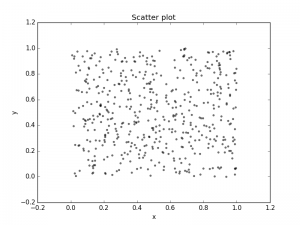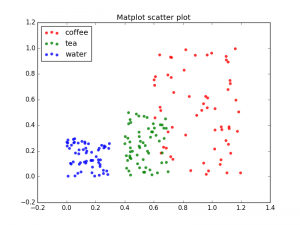# Matplotlib scatterplot

Python hosting: Host, run, and code Python in the cloud!

Matplot has a built-in function to create scatterplots called scatter(). A scatter plot is a type of plot that shows the data as a collection of points. The position of a point depends on its two-dimensional value, where each value is a position on either the horizontal or vertical dimension.

Related course

Scatterplot example
Example:Scatter plot created with Matplotlib

Scatter plot with groups
Data can be classified in several groups. The code below demonstrates that:

Related courseScatter plot with classes